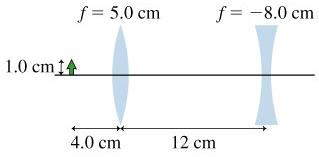# Problem: 1.) Find the position of the final image of the 1.0-cm-tall object2.) Find the size of the final image of the 1.0-cm-tall object3.) Is the object inverted or erect?

###### FREE Expert Solution

Lens equation:

$\overline{)\frac{\mathbf{1}}{\mathbf{f}}{\mathbf{=}}\frac{\mathbf{1}}{{\mathbf{s}}_{\mathbf{o}}}{\mathbf{+}}\frac{\mathbf{1}}{{\mathbf{s}}_{\mathbf{i}}}}$

Magnification:

$\overline{){\mathbf{m}}{\mathbf{=}}\frac{{\mathbf{h}}_{\mathbf{i}}}{{\mathbf{h}}_{\mathbf{o}}}{\mathbf{=}}{\mathbf{-}}\frac{{\mathbf{s}}_{\mathbf{i}}}{{\mathbf{s}}_{\mathbf{o}}}}$

(1.)

For the convex lens:

f = 5.0 cm

so = 4.0 cm

si = (sof)/(so - f) = (4.0 × 5.0)/(4.0 - 5.0) = -20 cm

For the concave lens:

f' = - 8 cm

82% (438 ratings)###### Problem Details1.) Find the position of the final image of the 1.0-cm-tall object

2.) Find the size of the final image of the 1.0-cm-tall object

3.) Is the object inverted or erect?